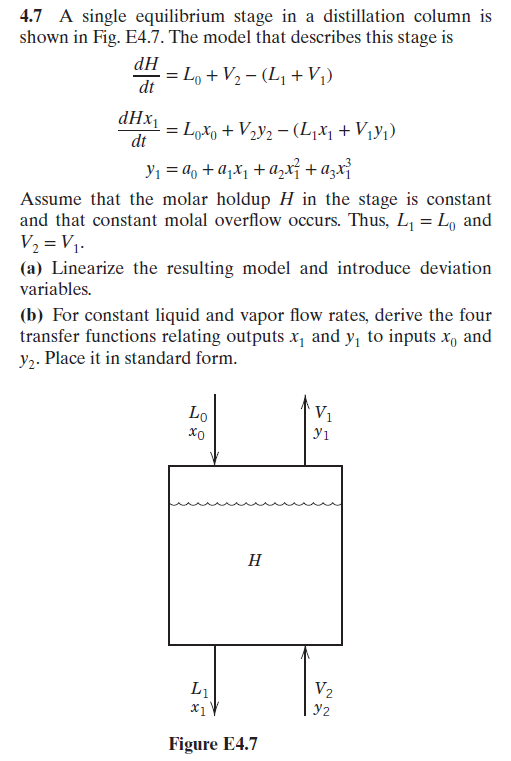4.7 A single equilibrium stage in a distillation column isshown in Fig. E4.7. The model that describes this stage isdtdHx1RA+44 +44 +a,Assume that the molar holdup H in the stage is constantand that constant molal overflow occurs. Thus, Li-L andV. =リ.(a) Linearize the resulting model and introduce deviationvariables.(b) For constant liquid and vapor flow rates, derive the fourtransfer functions relating outputs x and y, to inputs x andy2. Place it in standard form.0xoV1V.1Figure E4.7

Questionhelp_outlineImage Transcriptionclose4.7 A single equilibrium stage in a distillation column is shown in Fig. E4.7. The model that describes this stage is dt dHx1 RA +44 +44 +a, Assume that the molar holdup H in the stage is constant and that constant molal overflow occurs. Thus, Li-L and V. =リ. (a) Linearize the resulting model and introduce deviation variables. (b) For constant liquid and vapor flow rates, derive the four transfer functions relating outputs x and y, to inputs x and y2. Place it in standard form. 0 xo V1 V. 1 Figure E4.7 fullscreen
Step 1

Part (a)

Assume that the molar holdup (H) of the given stage is constant and the molar overflow which occurs is also constant. Therefore, Lo = L1 and V2 = V1. Thus, from the given first equation,

Step 2

Now, apply this in the second given equation as shown below.

Step 3

Apply Tylor series expansion on the given equation of y1 ...

Want to see the full answer?

See Solution

Want to see this answer and more?

Our solutions are written by experts, many with advanced degrees, and available 24/7

See Solution
Tagged in

Chemical Engineering